Practice Questions- Relations and Functions

# Practice Questions- Relations and Functions - Mathematics (Maths) Class 11 - Commerce

Ques 1: Find a and b if (a – 1, b + 5) = (2, 3)If A = {1,3,5}, B = {2,3} find : (Question-2, 3)
(i)  A × B
(ii) Let A = {1,2}, B = {2,3,4}, C = {4,5}
(iii) A × (B ∩ C)

(iv) A × (B ∪ C)
Ans: a = 3, b = -2
(i) A × B = {(1,2), (1,3), (3,2), (3,3), (5,2), (5,3)}
(ii) B × A = { (2,1), (2,3), (2,5), (3,1), (3,3), (3,5)}
(ii) {(1,4), (2,4)}
(iv) {(1,2), (1,3), (1,4), (1,5), (2,2), (2,3), (2,4), (2,5)}

Ques 2:  If P = {1,3}, Q = {2,3,5}, find the number of relations from A to B
Ans: 26 = 64

Ques 3: If A = {1,2,3,5} and B = {4,6,9}, R = {(x, y) : |x – y| is odd, x ∈ A, y ∈ B} Write R in roster form
(i) R = { (1,1), (2,2), (3,3), (4,4), (4,5)}
(ii) R = { (2,1), (2,2), (2,3), (2,4)}
Which of the following relations are functions. Give reason.
Ans:
R = { (1,4), (1,6), (2,9), (3,4), (3,6), (5,4), (5,6)}
(i) Not a function because 4 has two images.
(ii) Not a function because 2 does not have a unique image.

Ques 4: If A and B are finite sets such that n(A) = m and n(B) = K find the number of relations from A to B
Ans: Linen n(A) = n and n(B) = k
∴ n(A * B)= ∩(A) * ∩(B)= mk
∴ the number of subsets of A x B = 2mk
∵ n(A) =m, then the number of subsets of A = m2
Since every subset of A * B is a relation from A to B therefore the number of relations from A to B = 2mk

Ques 5: Let f = {(1,1),(2,3),(0,-1),(-1,3),........} be a function from z to z defined f(x) ax +b,  by for same integers a and b determine a and b.

Ans: Given f(x) ax +b
Since (1,1) ∈ f1f(1) = 1 ⇒ a+b = 1.......(i)
(2,3) ∈ f.f (2) = 3 ⇒ 2a+b =3........(ii)
Subtracting (i) from(ii) we set a=2
Substituting a = 2 is (ii) we get 2+b=1
⇒ b = -1
Hence a = 2, b = -1

Ques 6: Express {(x,y): y+ 2x =5, xy ∈ w}  as the set of ordered pairs
Ans:
Since and x, y ∈ w,
Put x=0, y+0= 5 ⇒ y=5
x=1, y+2*1= 5⇒ y =3
x=2,y+2*2=5 ⇒ y=1
For anther values of x∈w , we do not get y∈w
Hence the required set of ordered peutes is {(0'5),(1,3),(2,1)}

Ques 7: If A = {1,2}, find (A*A*A)
Ans:
A*A*A ={(1,1,1),(1,1,2),(1,2,1),(2,1,1),(2,2,1),(2,2,2)}

Ques 8: Let f = [(0,-5),(1,-2),(2,1),(3,4), (4,7)}j be a linear function from z into= find
Ans:
f(x) =3x -5

Ques 9: If the ordered pairs (x-2,2y +1) and (y- 1,x+2) are equal, find x&Y
Ans: x=3, y=2

Ques 10: Let A = {-1,2,5,8}, B= {o,1,3,6,7} and R be the relation, is one less than from A to B then find domain and Range of R
Ans: Given A= {-1,2,5,8}, B= {o,1,3,6,7} and R is the relation 'is one less than' from
A to B therefore R = [ (-1,0),(2,3),(5,6) ]
Domain of R = {-1,2,5} and range of R = {0,3,6}

Ques 11: Let R be a relation from N to N define by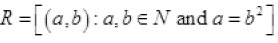.
Is the following true implies a,b ∈ R implies (b,a) ∈ R
Ans:
No; let a = 4, b = 2 As 4 = 22, so (4,2) ∈ but 2 ≠ 42 so (2,4) ∈ R

Ques 12: Let be the set of natural numbers and the relation R be define in N by  R =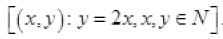what is the domain, co domain and range of ? Is this relation a function?

Ans: Given R =∴ Domain of R = N. co domain of R = N and Range of R is the set of even natural numbers.
Since every natural number x has a unique image 2x therefore, the relation R is a function.

Ques 13: 40.Let R = {(x,y): y = x +1} and y ∈{0,1,2,3,4,5} list the element of R
Ans:
R= {(-1,0),(0,1),(1,2),(2,3),(3,4),(4,5)}

Ques 14: Let f be the subset of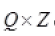defined by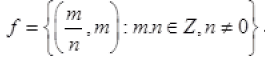is f a functionjustify your answer
Ans:
f Is not a function from Q to Z.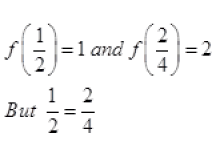∴ One element 1/2 have two images
∴ f is not function

Ques 15: The function 'f' which maps temperature in Celsius into temperature in Fahrenheit is defined by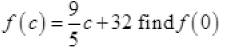Ans: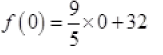f (o) = 32

Ques 16: If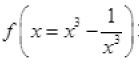Prove that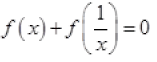Ans: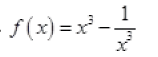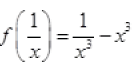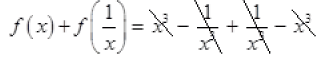= 0

Ques 17: If A and B are two sets containing m and n elements respectively how many different relations can be defined from A to B ?
Ans:
2m+n

Ques 18: Let A = {1,2,3,4}, B = {1,4,9,16,25} and R be a relation defined from A to B as, R = {(x, y) : x ϵ A, y ϵ B and y = x2}
(a) Depict this relation using arrow diagram.
(b) Find domain of R.
(c) Find range of R.
(d) Write co-domain of R.

Ans: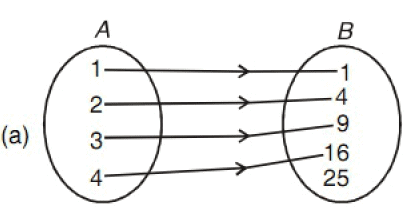(b) {1,2,3,4}
(c) {1,4,9,16}
(d) {1,4,9,16,25}

Ques 19: Let R = { (x, y) : x, y ϵ N and y = 2x} be a relation on N. Find :
(i) Domain
(ii) Codomain
(iii) Range Is    this    relation    a    function    from    N    to    N

Ans: (i) N
(ii) N
(iii) Set of even natural numbers yes, R is a function from N to N.

Ques 20: Find the domain and range of, f(x) = |2x – 3| – 3
Ans:
Domain is R
Range is [–3, ∞)

Ques 21: Draw the graph of the Constant function, f : R ϵ R; f(x) = 2 x ϵ R. Also find its domain and range.
Ans:
Domain = R
Range = {2}

Ques 22: Let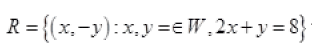Then
(i) Find the domain and the range of R
(ii) Write R as a set of ordered pairs.
Ans:
(i) Given 2x + y = 8 and x y ϵ w
Put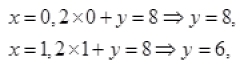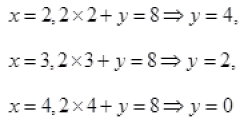for all the values x y ϵ w we do not get y ϵ w
∴ domain of R = {0,1,2,3,4} and range of R = {8,6,4,2,0}
(ii) R as a set of ordered pairs can be written as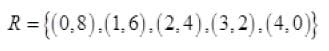The document Practice Questions- Relations and Functions | Mathematics (Maths) Class 11 - Commerce is a part of the Commerce Course Mathematics (Maths) Class 11.
All you need of Commerce at this link: Commerce

## FAQs on Practice Questions- Relations and Functions - Mathematics (Maths) Class 11 - Commerce

 1. What is a relation in mathematics?A relation in mathematics is a set of ordered pairs that establishes a connection between two sets. It describes how elements from one set relate to elements from another set.
 2. What are the types of relations?There are several types of relations in mathematics, including: - Reflexive relation: Every element is related to itself. - Symmetric relation: If a is related to b, then b is related to a. - Transitive relation: If a is related to b and b is related to c, then a is related to c. - Equivalence relation: A relation that is reflexive, symmetric, and transitive. - Function: A relation where each input has a unique output.
 3. What is the difference between a function and a relation?A function is a specific type of relation where each input has a unique output. In other words, for every element in the domain, there is exactly one element in the codomain that it is related to. A relation, on the other hand, simply describes how elements from one set relate to elements from another set without the requirement of uniqueness.
 4. How do you determine if a relation is a function?To determine if a relation is a function, you can use the vertical line test. If every vertical line intersects the graph of the relation at most once, then the relation is a function. Alternatively, you can check if each input in the relation has a unique output. If there are no repeated outputs, the relation is a function.
 5. Can a relation be both symmetric and asymmetric?No, a relation cannot be both symmetric and asymmetric simultaneously. A symmetric relation implies that if a is related to b, then b is related to a. On the other hand, an asymmetric relation implies that if a is related to b, then b cannot be related to a. These two conditions contradict each other, so a relation cannot satisfy both symmetric and asymmetric properties at the same time.

## Mathematics (Maths) Class 11

157 videos|210 docs|132 tests

## Mathematics (Maths) Class 11

157 videos|210 docs|132 tests
Signup to see your scores go up within 7 days! Learn & Practice with 1000+ FREE Notes, Videos & Tests.
10M+ students study on EduRev
Track your progress, build streaks, highlight & save important lessons and more!(Scan QR code)
Related Searches

,

,

,

,

,

,

,

,

,

,

,

,

,

,

,

,

,

,

,

,

,

;